Learning Library

Understanding Decimals

Working with decimals can be difficult, but these worksheets will help break 'em down. With a little practice your fifth grader with become a master of decimal math.

Click on a worksheet in the set below to see more info or download the PDF.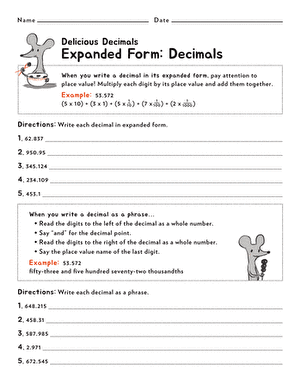Expanded Form: Decimals

Children practice writing decimals in their expanded form and as phrases in this practice worksheet.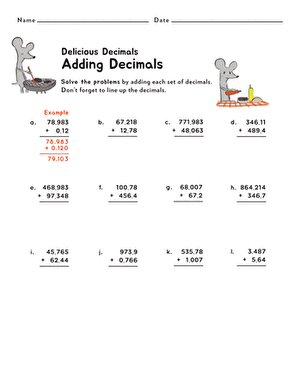Children practice adding integers and decimals to the thousandths place in this practice worksheet.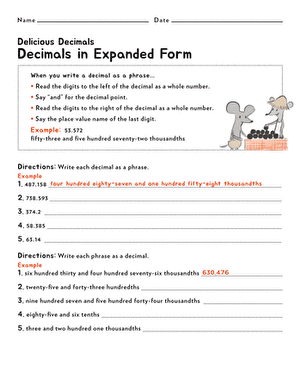Decimals in Expanded Form

Children learn to write decimals as phrases and phrases as decimals with this practice worksheet.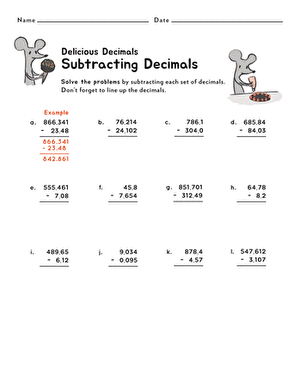Subtracting Decimals

Time for decimal practice! Children subtract sets of decimals in this practice worksheet.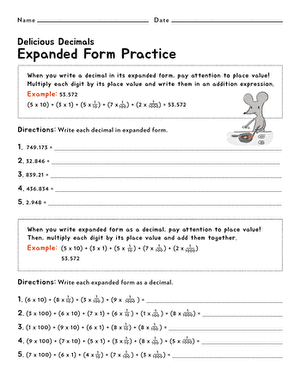Expanded Form

Children find tips for writing decimals in expanded form—and expanded form as a decimal—in this useful practice worksheet.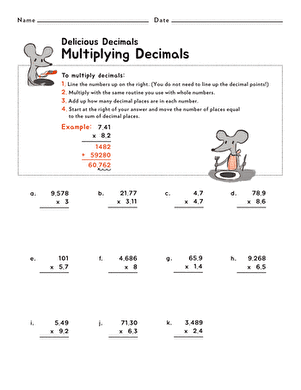Multiplying Decimals

Children learn the steps for multiplying decimals with this easy-to-follow tutorial and practice sheet.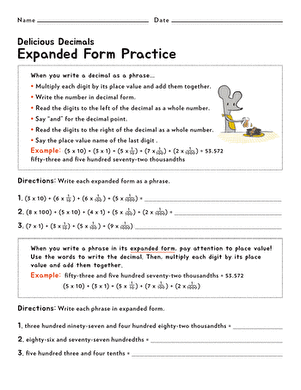Expanded Form Practice

Children practice writing expanded form decimals as phrases—and phrases as expanded form decimals—in this math worksheet.Multiplying Numbers with Decimals

If your child needs a little help with decimal multiplication, here's the worksheet for her.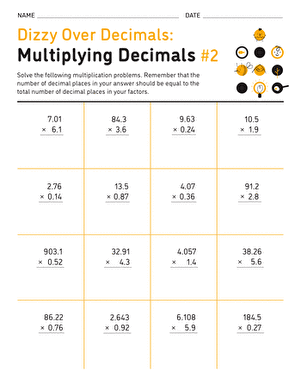Dizzy Over Decimals: Multiplication #2

Here's a sheet that's sure to give your child all the practice he needs with decimal multiplication.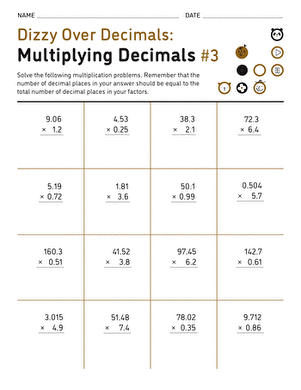Dizzy Over Decimals: Multiplication #3

This math practice sheet is chock-full of decimal multiplication problems that are guaranteed to help your child get more comfortable with his schoolwork.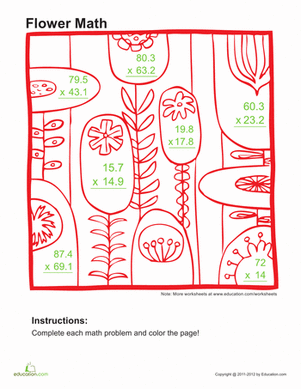Multiply Decimals and Color 1

Practice multiplying decimals with a cute coloring page! Your child can work out some tough three-digit equations involving decimals as she colors.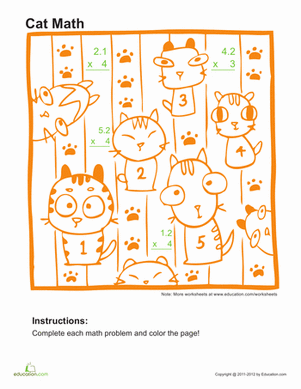Multiply Decimals and Color 3

Give your student a break from boring textbook problems, and practice multiplying decimals with a coloring page!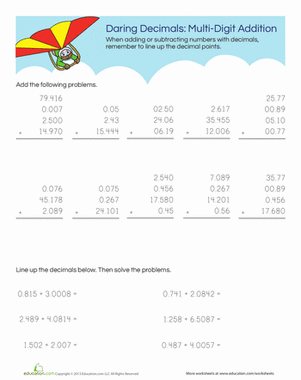Daring Decimals: Multi-Digit Addition

The key to adding with decimals is lining up the decimal points. Help your fifth grader practice decimal addition with carrying.

Want to download this whole set as a single PDF?

Create new collection

0

New Collection>

0 items

What could we do to improve Education.com?

Please note: Use the Contact Us link at the bottom of our website for account-specific questions or issues.

What would make you love Education.com?